## Figures index

#### Mbujje Joel Webster, Wei XiaoSheng, Makorogo Javilla Barugahare

American Journal of Civil Engineering and Architecture. 2016, 4(4), 125-132 doi:10.12691/ajcea-4-4-3• Figure 1. Typical development with time of (a) yield stress (b) electrical resistivity and rate of resistivity• Figure 2. Algorithm to predict yield stress from electrical resistivity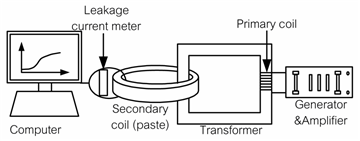• Figure 3. Schematic of Non Contacting Electrical Resistivity Machine (NC-ERM)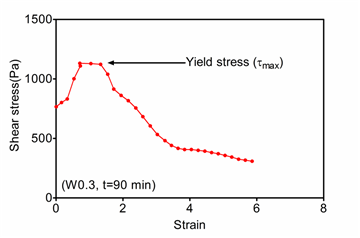• Figure 4. Typical shear stress with time (w/c=0.3, t=90 min)• Figure 5. Yield stress against time from a rheometer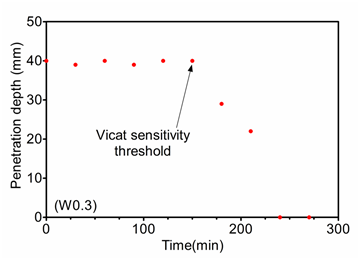• Figure 6. Depth of penetration with time (w/c=0.3)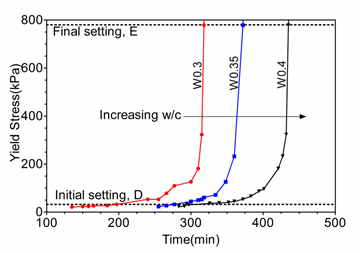• Figure 7. Yield stress with time from vicat needle penetration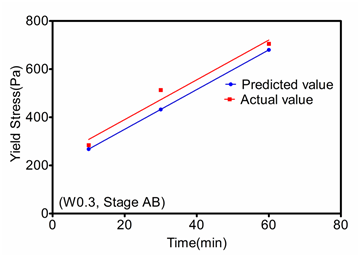• Figure 8. Electrical resistivity development with time for different cement pastes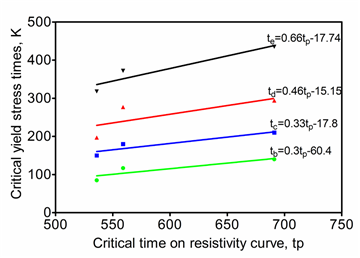• Figure 9. Rate of Resistivity development with time for different cement pastes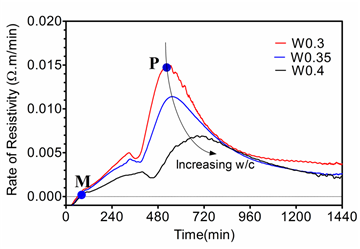• Figure 10. Linear regression of yield stress critical times, K with tp• Figure 11. Validation of model in the stage AB (w/c=0.3)